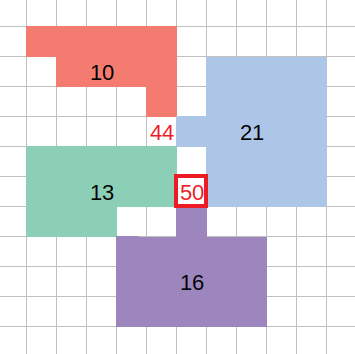Leetcode 827：最大人工岛（超详细的解法！！！）
in leetcode with 0 comment

输入: [[1, 0], [0, 1]]

输入: [[1, 1], [1, 0]]

输入: [[1, 1], [1, 1]]

• 1 <= grid.length = grid.length <= 50
• 0 <= grid[i][j] <= 1class Solution:
def largestIsland(self, grid):
r, c = len(grid), len(grid)

def check(x, y):
if x < 0 or x >= r or y < 0 or y >= c:
return 0
return 1

def dfs(x, y, color):
if not check(x, y) or grid[x][y] != 1:
return 0
res = 1
grid[x][y] = color
for i, j in [[1, 0], [-1, 0], [0, 1], [0, -1]]:
res += dfs(x + i, y + j, color)
return res

data, res, color = [0, 0], 0, 2
for i in range(r):
for j in range(c):
if grid[i][j] == 1:
data.append(dfs(i, j, color))
color += 1

for i in range(r):
for j in range(c):
if grid[i][j] == 0:
colors = set(grid[x+i][y+j] for x, y in [[1, 0], [-1, 0], [0, 1], [0, -1]] if check(i + x, j + y))
res = max(res, sum(data[color] for color in colors) + 1)
return r*c if res == 0 else res

「如果我的文章对你有很大帮助，那么不妨～！」Responses